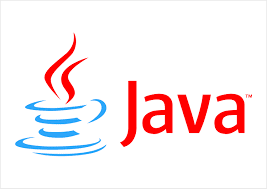# Java8 - Learn Lambda Expressions in 10 minutes with tutorials and examples

In this blog post, I will cover the basics of java8 Lambda expressions tutorials with examples.

### Lamda Expressions IntroductionLamda Expresesions are important feature introduced in java8.

This expression enables to create an anonyms class with implementation of FInterfacesinterfaces. Anonyms class has no name for it.

The functional interface contains only single abstract methods, introduced in java8 to achieve functional programming in java language. Please see my previous articles on Functional Interfaces. Another way is to represent functional programming with methods of a class using method reference. Please see my previous post about Method reference.

#### Java7  Lambda Expression, prior to Java 8

Prior to java8, without lambda expression, We are going to create a  anonyms class for interface implementation.

``````public class AnonymousClassesExample {
public static void main(String[] args) {
MathOperation mo = new MathOperation() {
@Override
public int add(int a, int b) {
return (a + b);
}
};
}
}
interface MathOperation {
public int add(int a, int b);
}
``````

output is

``````4

``````

#### Java8 Lamda Expression Example

The same functionality is rewritten with a lambda expression. Amount of Code is reduced.

``````public class LamdaExpressionExample {
public static void main(String[] args) {
MathOperation math=(a,b)->(a + b);
}

}
@FunctionalInterface
interface MathOperation {
public int add(int a, int b);
}

``````

Output is

``````4

``````

### Syntax

Lambda Expression can be created using the following syntax. It uses an arrow operator which contains hyphen followed by greater than a symbol. The left side of the arrow operator contains parameters. The right side of the arrow operator have expression or statements

``````(Parameters) --> expression or
(Parameters) --> {statements;}

``````

Parameters – These all or empty or nonempty values of left side arrow operator Arrow Operator symbol expression - if this specified, the semicolon is not required and curly braces optional Statements - if there are more than one statements, must be enclosed in curly braces. and need to add a semicolon at end of statement This contains expressions/body of the lambda expression

#### Zero Parameter

``````() -> System.out.println("Lambda Expression without Arguments");

``````

#### One Parameter

``````(value) --> System.out.println("Lambda Expression with one Arguments"+value);

``````

#### Multiple Parameter

``````(value1, value2) --> System.out.println("Lambda Expression with one Arguments"+value1+value2);

``````

### Features

These are functions without a name - Anonyms functions
The body contains zero or more statements. If it is a single statement, curly braces are not required. if more than one statements, These should be enclosed in curly braces
Return keyword is not required by default. The return type of anonymous function is the same as the value type of the statements.
These are mapped to Functional Interfaces.

Allows  Functional Programming via the Functional interface.
The reduced line of code This combines with streams API is more efficient during a parallel iteration of data structures

• It is hard to debug when an error occurs
• Difficulty to understand the code easily

### Lamda Expression Examples

The below section contains frequently used lambda expression usage examples  Lamda Expression without arguments Example Lamda Expression is defined without arguments. The functional interface has only one abstract method. @FunctionalInterface annotations are optional and not required.

``````public class LamdaExpressionWithoutParametersExample {
public static void main(String[] args) {
Message msg = () -> {
return "Hi How are you?";
};
System.out.println(msg.getMessage());
}
}
@FunctionalInterface
interface Message {
public String getMessage();
}
``````

Output is

``````Hi How are you?

``````

Lamda Expression Single Argument Example Here single argument lamda expression is defined. arguments can be either enclosed in parenthesis.

``````public class LamdaExpressionWithParametersExample {
public static void main(String[] args) {
// Lamda expression single argument enclosed in braces
MathOperation math = (value) -> {
return value + 1;
};
// Lamda expression single argument without braces
MathOperation math1 = value -> {
return value + 1;
};
System.out.println(math.incrementByOne(1));
System.out.println(math1.incrementByOne(4));

}

}

interface MathOperation {
public Integer incrementByOne(Integer i);

}

``````

Output is

``````2
5

``````

#### Lamda Expression Two Arguments Example

This example contains the usage of various use cases

1. Lamda Expression defined with two arguments, argument type is not declared in this, the type is inferred from the value of the argument.
2. Lamda Expression defined with two arguments their type included.
3. Lamda Expression defined without the return keyword. When return keyword is not defined, Curly braces is not required.
``````public class LamdaExpressionWithParametersExample {
public static void main(String[] args) {
// Lamda expression two argument without type of argument
MathOperation math = (v1, v2) -> {
return v1 + v2;
};
// Lamda expression two arguments with type of argument
MathOperation math1 = (Integer v1, Integer v2) -> {
return v1 + v2;
};
// Lamda expression two arguments without return type
MathOperation math2 = (Integer v1, Integer v2) -> (v1 + v2);

}
}

interface MathOperation {
public Integer add(Integer v1, Integer v2);

}

``````

Output is

``````17
48
76

``````

#### For Each Example

This is an example forEach of list objects or primitive types

``````List list = new ArrayList();
list.forEach((value) -> System.out.println(value));
``````

Output is

``````1
6
8

``````
Similar Posts
Subscribe
You'll get a notification every time a post gets published here.

Related posts